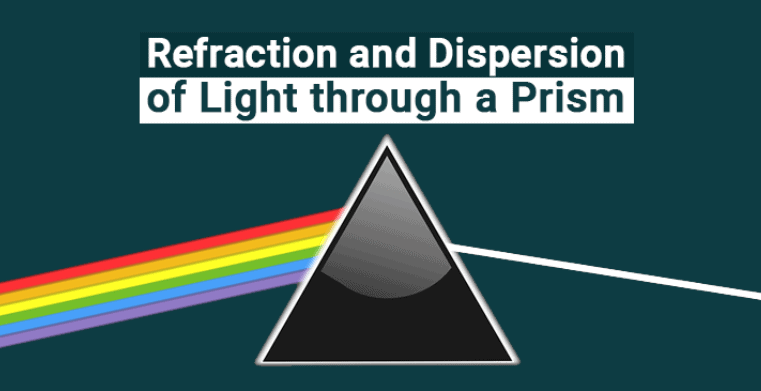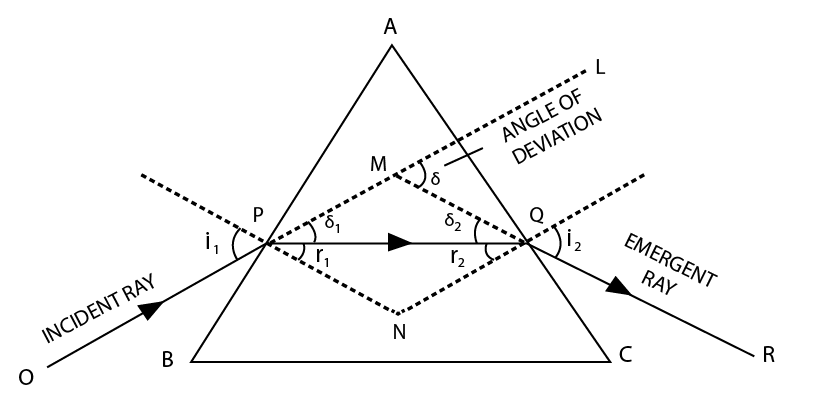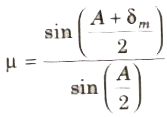Courses

# Prism and Dispersion by a Prism Class 12 Notes | EduRev

## Class 12 : Prism and Dispersion by a Prism Class 12 Notes | EduRev

The document Prism and Dispersion by a Prism Class 12 Notes | EduRev is a part of the Class 12 Course Physics For JEE.
All you need of Class 12 at this link: Class 12

What is a Prism?

• Prism is a uniform transparent medium bounded between two refracting surfaces, inclined at an angle.
• Change in the direction of propagation of light when passed through the different medium is called refraction of light.
• The splitting of white light into seven constituent colours takes place when passed through the prism. This is known as the dispersion of light by a prism.Dispersion of Light Through Prism

• With the help of a narrow beam of light, a glass prism, and a white wall it is possible to produce the band of seven colors using white light. Keep this arrangement near the window. Place the glass prism in such manner that the sunlight through the window falls on one side of the prism and then on the white wall.
• You can see that the light reflected on the wall has several colors. The prism splits the white light into seven different colors. This splitting of white light into many colors is called as a dispersion of light. Dispersion is nothing but splitting of white light into its constituents colors.i.e into seven different colors.
• The seven colors are violet, indigo, blue, green, yellow, orange, and red (VIBGYOR). The pattern of color which is obtained is called as a spectrum.  Sometimes in the rainbow, you may not see all the seven colors. This is because of the colors overlap each other.

Refraction Of Light Through Prism

• When light travels from one medium to another, the speed of its propagation changes, as a result, it ‘bends’ or is ‘refracted’. Now when light passes through a prism, it is refracted towards the base of the triangle.
• The different colours in the spectrum of light have different wavelengths. Therefore, the speed with which they all bend varies depending on this wavelength, where violet bends the most, having the shortest wavelength and red bends the least, having the longest wavelength.
• Because of this, the dispersion of white light into its spectrum of colours takes place when refracted through a prism.
• Angle of Deviation: The angle subtended between the direction of incident light ray and emergent light fay from a prism is called angle of deviation (δ).• Let A, B, C be the glass of the prism. Suppose BC is the base and AB and AC are its two refracting surfaces. From the above figure, we can say that OP is the incident. The ray traveling through the rarer medium and than the refractive index of the prism is the incident ray. As the ray PQ strikes the surface of the and it is called as the refracted ray. OR is the emergent ray which comes out.

• When the ray light enters the glass, it bends towards normal and when ray comes out, it bends away from the normal. Now the angle between the emergent ray and incident ray is the angle of deviation. For a single refracting surface,  δ = |i – r|
In this case, δ = (i1 + i2) – (r1 + r2)
δ = i1 + i2 – A, A is the angle between the prism between two lateral surfaces.

• We know that ∠A and ∠Q is 180º and Angle of the prism of (A) is: r1 + r2
r1  is the angle of refraction inside the prism and r2 is the angle of refraction outside it. For an angle of minimum deviation, δ is minimum and i1 = i2 = i
δmin = 2i – A
For small A, δ = (µ – 1) A

• Minimum Angle of Deviation for a Prism: At the minimum deviation, Dm the refracted ray inside the prism becomes parallel to its base, i.e. i = e ⇒ r1 = r2 = r, then r = A/2 and Dm = 2i – A, where i is the angle of emergence, r1 and r2 are the angles of refraction and A is the angle of the prism.

• Prism Formula
The refractive index of the material of prism:• Angular Dispersion: The angle subtended between the direction of emergent violet and red rays of light from a prism is called angular dispersion.
Angular dispersion
(θ) δv – δR = (μv – μR)A
where δv and δR are angle of deviation.

• Dispersive Power
W = θ/δY = (μv – μ R) / (μY – 1)
where μY = (μ v + μ R ) / 2, is mean refractive index.

Offer running on EduRev: Apply code STAYHOME200 to get INR 200 off on our premium plan EduRev Infinity!

## Physics For JEE

187 videos|516 docs|263 tests

,

,

,

,

,

,

,

,

,

,

,

,

,

,

,

,

,

,

,

,

,

;